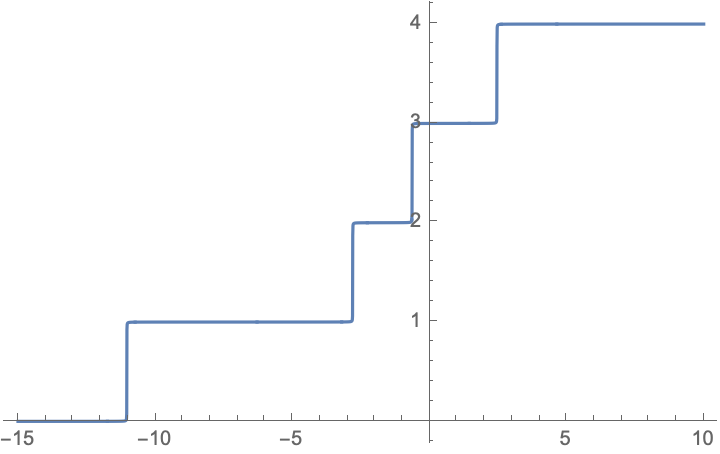# Counting eigenvalues without diagonalizing a matrix

Today's arXiv has a paper by Pierpaolo Vivo, Index of a matrix, complex logarithms, and multidimensional Fresnel integrals, which asks the question whether it is possible to calculate the number $$N(\lambda_1,\lambda_2)$$ of eigenvalues of a real symmetric matrix $$M$$ that lie in the interval $$(\lambda_1,\lambda_2)$$ , without explicitly diagonalizing the matrix. The author points out that a certain formula in the literature based on the branch-cut structure of the complex logarithm generically fails to produce the correct result.

In a discussion with my colleagues (thank you, Fabian & Inanc) we could fix this branch-cut ambiguity by first differentiating the logarithm and then integrating, $$N(\lambda_1,\lambda_2)=\frac{1}{2\pi i}\lim_{\epsilon\rightarrow 0^+}\int_{\lambda_1}^{\lambda_2}d\lambda\; \frac{d}{d\lambda}\left[\log\det(M-\lambda+i\epsilon)-\log\det(M-\lambda-i\epsilon)\right].$$ As an example, applied to the matrix $$M$$ defined in equation 3 of the cited paper this gives the following plot for $$N(-15,\lambda)$$ as a function of $$\lambda$$, in agreement with the eigenvalues at $$-11.03, -2.80, -0.63, 2.464$$.Question: From a computational point of view, this method to count levels is unlikely to be efficient relative to a direct diagonalization. The cited paper develops a method based on Fresnel integrals. Is there an alternative more efficient approach?

I note an earlier MO question along the same lines, but for the specific case of a random matrix with localized eigenstates; here the idea is to have a method that works generically.
• Take a look at FEAST-type algorithms; I am not an expert, but I think they do something similar to what you suggest. Nov 26, 2020 at 7:16

First of all, I must quote that diagonalizing $$M$$ is not a method, because there is no explicit way to carry this out. It amounts to calculating the roots of a polynomial ! At best, one can do this in an approximate way.
Instead, I suggest to perform a preliminary step : put $$M$$ in Hessenberg form. This is done (method of Givens) in $$O(n^3)$$ operations, by using rotations in coordinate planes. This calculation is stable in the sense that the rounding errors, if any, are not amplified, so the spectrum is not polluted by more than the elementary rouding error of the machine.
Because $$M$$ was symmetric, its Hessenberg form $$H$$, still symmetric, is actually tridiagonal. Then you can form a Sturm sequence by taking the principal submatrices of $$H$$ and their characteristic polynomials $$P_j$$. Their calculation is extremely cheap in the tridiagonal case: say that $$H=\begin{pmatrix} a_1 & b_1 & 0 & \cdots & 0 \\ b_1 & \ddots & \ddots & \ddots & \vdots \\ 0 & \ddots & \ddots & \ddots & 0 \\ \vdots & \ddots & \ddots & \ddots & b_{n-1} \\ 0 & \cdots & 0 & b_{n-1} & a_n \end{pmatrix}.$$ Then $$P_n(X)=(X-a_n)P_{n-1}(X)-b_{n-1}^2P_{n-2}(X).$$ We may assume that the $$b_j$$'s are non-zero (otherwise, treat matrices of smaller sizes). Then $$(P_j(c)=0)\longrightarrow(P_{j+1}(c)P_{j-1}(c)<0),$$ the Sturm assumption.
Now Sturm Theorem gives you the number of eigenvalues in $$[a,b]$$ as the difference $$V(a)-V(b)$$, where $$V(x)$$ is the number of sign changes in the sequence $$(P_n(x),\ldots,P_0\equiv1)$$.
• @korrok . You comment seems irrelevant. I don't speak of an algorithm which would calculate an (approximation of) an eigenvalue, and then continue to the next one. I speak of Givens's algorithm which, in $O(n^3)$ operations (thus is exact modulo rounding errors), puts a matrix $M$ into a Hessenberg form. Nov 26, 2020 at 5:35